Array in C can be defined as a method of clubbing multiple entities of similar type into a larger group. These entities or elements can be of int, float, char, or double data type or can be of user-defined data types too like structures. However, in order to be stored together in a single array, all the elements should be of the same data type.  The elements are stored from left to right with the left-most index being the 0th index and the rightmost index being the (n-1) index.

#### Post Graduate Program: Full Stack Web Development

in Collaboration with Caltech CTMEEnroll Now

Array  in C are of two types; Single dimensional arrays and Multidimensional arrays.

• Single Dimensional Arrays: Single dimensional array or 1-D array is the simplest form of arrays that can be found in C. This type of array consists of elements of similar types and these elements can be accessed through their indices.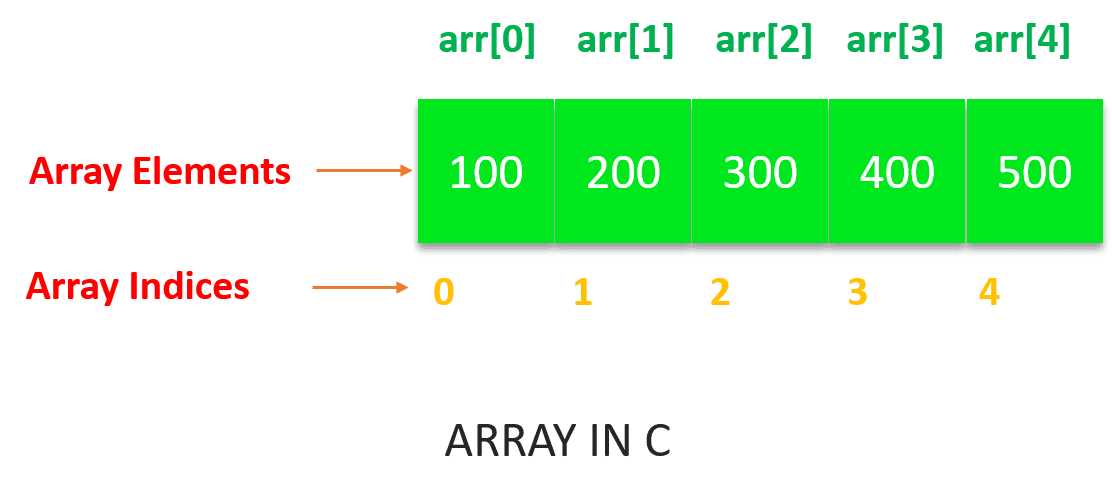• Multi-dimensional Arrays: The most common type of multi-dimensional array that is used in the C language is a 2-D array. However, the number of dimensions can be more than 2 depending upon the compiler of the user’s system. These arrays consist of elements that are array themselves.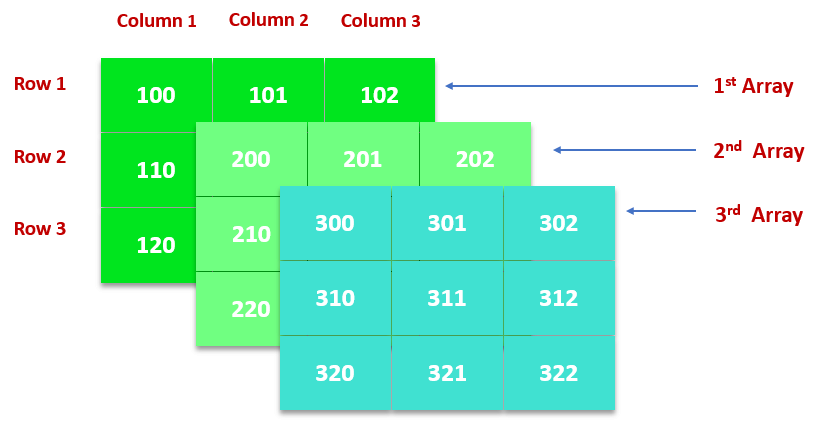## Why Do We Need Arrays?

If we have a small number of elements, let us say we want 3 variables, then we can declare them separately like var1, var2, and var3. But if we have a large number of variables then we can use arrays to store them.

Let us take a real-life example. Suppose you want to make a program that prints 1-100 digits. Now in C language, you can achieve this by 2 methods. The first one is to make 100 variables and store the numbers from 1-100 in those variables separately and then print each digit. The second method is to create an array of size 100 and store the numbers in that array using a loop. These digits can be printed using a single loop in linear complexity. It is clear that the second method is more optimized and desirable than the first one as it is more convenient to store these values in a single array rather than creating 100 variables.

## Declaration and Initialization of Array in C

There are various ways in which an array can be declared and initialized in various ways. You can declare an array of any data type (i.e. int, float, double, char) in C. The following ways can be used to declare and initialize an array in C.

• ### Array Declaration by Specifying the Size

Arrays can be declared by specifying the size or the number of array elements. The size of the array specifies the maximum number of elements that the array can hold. In the latest version of C, you can either declare an array by simply specifying the size at the time of the declaration or you can provide a user-specified size. The following syntax can be used to declare an array simply by specifying its size.

// declare an array by specifying size in [].

int my_array1;

char my_array2;

// declare an array by specifying user defined size.

int size = 20;

int my_array3[size];

When an array is declared without allocating any value, then it stores a garbage value. If you access any uninitialized array value, then just like any uninitialized variable, it will give you a garbage value.

• ### Array Declaration by Initializing Elements

An array can be initialized at the time of its declaration. In this method of array declaration, the compiler will allocate an array of size equal to the number of the array elements. The following syntax can be used to declare and initialize an array at the same time.

// initialize an array at the time of declaration.

int my_array[] = {100, 200, 300, 400, 500}

In the above syntax, an array of 5 elements is created and even though the array size has not been specified here, the compiler will allocate a size of 5 integer elements.

• ### Array Declaration by Specifying the Size and Initializing Elements

An array can also be created by specifying the size and assigning array elements at the time of declaration. This method of array creation is different from the previous one. Here, if the number of initialized elements is less than the size of the array specified, then the rest of the elements will automatically be initialized to 0 by the compiler. See the following syntax to understand this.

// declare an array by specifying size and

// initializing at the time of declaration

int my_array1 = {100, 200, 300, 400, 500}; // my_array1 = {100, 200, 300, 400, 500}

//

int my_array2 = {100, 200, 300};  // my_array2 = {100, 200, 300, 0, 0}

In the above array syntax, my_array1 is an array of size 5 with all five elements initialized. Whereas, my_array2 is an array of size 5 with only three of its elements initialized. The remaining two elements of the second array will be initialized to 0 by the compiler.

#### New Course: Full Stack Development for Beginners

Learn Git Command, Angular, NodeJS, Maven & MoreEnroll Now• ### Array Initialization Using a Loop

An array can also be initialized using a loop. The loop iterates from 0 to (size – 1) for accessing all indices of the array starting from 0. The following syntax uses a “for loop” to initialize the array elements. This is the most common way to initialize an array in C.

// declare an array.

int my_array;

// initialize array using a “for” loop.

int i;

for(i = 0; i < 5; i++)

{

my_array[i] = 2 * i;

}

// my_array = {0, 2, 4, 6, 8}

In the above syntax, an array of size 5 is declared first. The array is then initialized using a for loop that iterates over the array starting from index 0 to (size – 1).

## Access Array Elements

Since an array is stored contiguously in the memory, it has indices starting from ”0” to “array_size – 1”, also known as zero-based indexing. This indexing represents the position in the array.

The array indices are used to access any element of the array in the following way:

array_name[index]

The index of the element to be accessed is specified within square brackets “[]”. The range of the index is- integers in the range [0, size).

Examples:

int my_array;

// access 1st element

my_array = 100;

// access 4th element

my_array = 300;

// access last element

my_array = 600;

## Input and Output Array Elements

Array values can be stored by taking input from the user and storing them in the array. The following example illustrates this:

// input an integer element and store it

// in 1st position of the array

​scanf(“%d”, &my_array);

// input a float element and store it

// in ith position of the array

scanf(“%f”, &my_array[i-1]);

Similarly, array elements can also be displayed in the output using the printf() method. The index is specified indicating the position of the element to be printed. The following example illustrates this:

// print the element stored at 1st position or 0th index

printf(“%d”, my_array);

// print the element stored at ith position or (i – 1)th index

printf(“%d”, my_array[i-1]);

## Advantages of Array in C

Arrays have a great significance in the C language. They provide several advantages to the programmers while programming. Some of them are:

• Arrays make the code more optimized and clean since we can store multiple elements in a single array at once, so we do not have to write or initialize them multiple times.
• Every element can be traversed in an array using a single loop.
• Arrays make sorting much easier. Elements can be sorted by writing a few lines of code.
• Any array element can be accessed in any order either from the front or rear in O(1) time.
• Insertion or deletion of the elements can be done in linear complexity in an array.

#### Full Stack Web Developer Course

To become an expert in MEAN StackView Course## Disadvantages of Array in C

Every advantageous thing comes with some disadvantages as well. This stands true for arrays as well. Below are some of the disadvantages of the array in C:

• Accessing an array out of bounds: The first disadvantage of arrays is that they are statically allocated. This means that once their size is initialized, it can not be increased or decreased. To understand this point, consider the example given below:

#include <stdio.h>

int main()

{

//declaring the array of size 20

int my_array;

//initialising the array elements

for (int i = 0; i < 20; i++)

{

//i will be the value of e

//very ith element of the array

my_array[i] = i;

}

// Print value at index 5 of the array

printf(“Element at index 5”

” is %dn”,

my_array);

// Print value at index 13 of the array

printf(“Element at index 13”

” is %dn”,

my_array);

// Print value at index 21 of the array

printf(“Element at index 21”

” is %d”,

my_array);

return 0;

}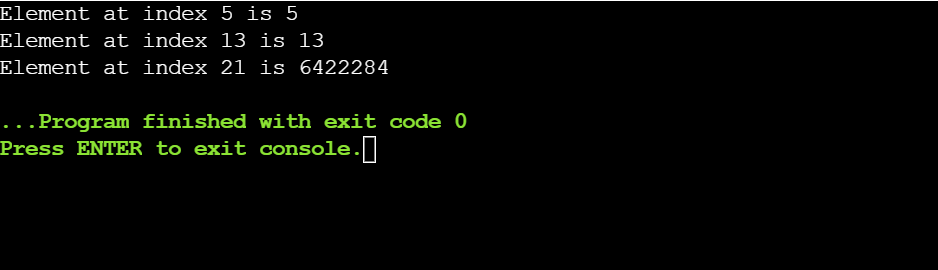In the above example, the initial value of the array arr is 20, so by printing the value of elements up to the index 20, we are getting the desired output. But when we try to print the element at the 21st index, it is giving a garbage value. This is because the array was accessed out of the bound index.

This issue can be resolved using malloc() and calloc() functions. These functions allocate the memory dynamically. We have a free() function that allows us to free the unwanted memory at our will. The below example illustrates the same:

#include <stdio.h>

#include <stdlib.h>

int main()

{

//*ptr will be storing the base

int *ptr;

int size, i;

// size of the array will be 5

size = 5;

printf(“Size of the array is: %dn”, size);

// allocating the array memory

//dynamically using malloc()

ptr = (int *)malloc(size * sizeof(int));

// Checking whether the memory has

//been successfully allocated by malloc

if (ptr == NULL)

{

printf(“Memory has not been allocated allocatedn”);

exit(0);

}

else

{

// Memory has been successfully allocated

printf(“Memory has been allocated successfullyn”);

// initializing the array elements

for (i = 0; i < size; ++i)

{

ptr[i] = i + 1;

}

// Print the elements of the array

printf(“The elements of the array are: “);

for (i = 0; i < size; ++i)

{

printf(“%d “, ptr[i]);

}

}

return 0;

}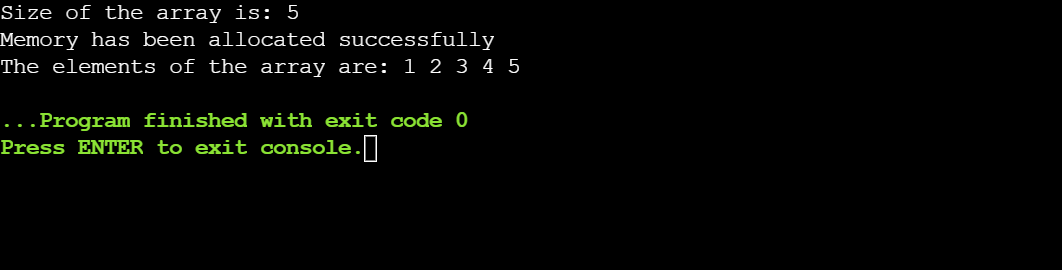#### FREE Java Certification Training

Learn A-Z of Java like never beforeEnroll Now• Homogeneity: We can store only a single type of element in the array i.e., arrays are homogeneous. We can not use it as a template. For example, if the array data type is char, then only characters can be stored in the array. If you try to store integers or any other element of a different data type, it will throw an error. To understand this point, consider the example given below:

#include <stdio.h>

int main()

{

// such declaration will throw

// Compilation Error

int my_array = {1, 2, “mango”, 4, 5, 6.2};

int i;

printf(“Elements of the array are: “);

for (i = 0; i < 6; i++)

{

printf(“%d “, my_array[i]);

}

return 0;

}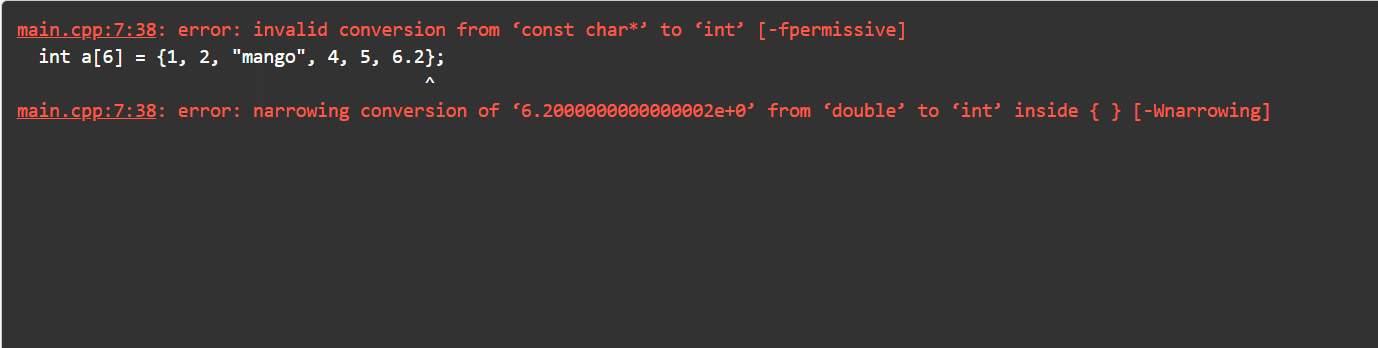In the above example, the data type of the array is int. But when we try to declare string and float values to the array, it throws a compilation error.

This issue can be resolved by creating a structure to store heterogeneous (non-homogeneous) values. Consider the below example to understand this concept:

#include <stdio.h>

// create a structure

struct example

{

int fruit_quant;

float fruit_rate;

char fruit_name;

};

int main()

{

// s1 – object of the structure

struct example s1 = {10, 90.45, “Mango”};

// accessing structure members

// using structure object

printf(“%dn”, s1.fruit_quant);

printf(“%fn”, s1.fruit_rate);

int i;

for (i = 0; s1.fruit_name[i] != ‘’; i++)

{

printf(“%c”, s1.fruit_name[i]);

}

return 0;

}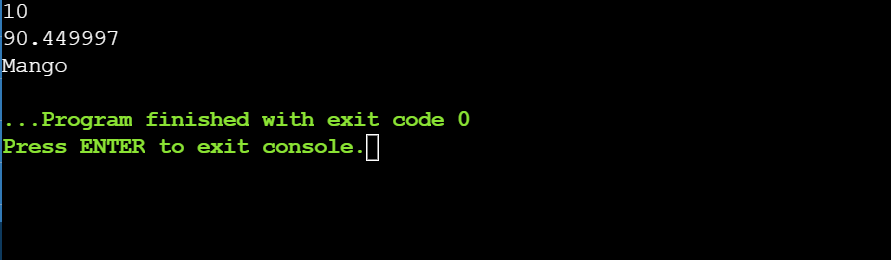## Examples of the 1-D Array in C

The following programs illustrate declaration, initialization, input/output operations, and basic operations like insertion,  deletion, sorting, and searching in the 1-D array in C.

### Example 1: Array Declaration, Input, and Output

#include <stdio.h>

int main()

{

// declare an array.

int my_array;

printf(“Enter array elements:n”);

// input array elements.

int i;

for (i = 0; i < 6; i++)

{

scanf(“%d”, &my_array[i]);

}

printf(“nArray elements are:n”);

// print array elements.

for (i = 0; i <= 5; i++)

{

printf(“%d “, my_array[i]);

}

return 0;

}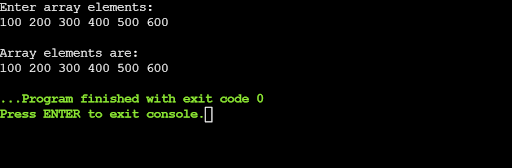In the above example, an integer array of size 6 is declared. This array can hold at most 6 integer elements. A “for loop” is used to input the array elements from the user. Similarly, a “for loop” is used to print these elements in the output. Both times the loop runs from 0 to 5, to iterate over all the array elements.

#### Full Stack Java Developer Course

In Partnership with HIRIST and HackerEarthEXPLORE COURSE### Example 2: Insertion and Deletion in an Array.

#include <stdio.h>

int main()

{

int i;

// initialize an array.

int my_array = {11, 6, 10, 50, 32, 56, 15, 98, 43, 22};

// input index where the element is to be inserted.

int pos;

printf(“Enter the position: “);

scanf(“%d”, &pos);

// input the element to be inserted.

int element;

printf(“Enter the element: “);

scanf(“%d”, &element);

if (pos > 10)

{

printf(“Input is invalid !”);

}

else

{

// right shifting array elements.

for (i = 9; i >= pos – 1; i–)

my_array[i + 1] = my_array[i];

// insert the element at “pos”.

my_array[pos – 1] = element;

printf(“Array after insertion is:n”);

// print array elements.

for (i = 0; i <= 10; i++)

printf(“% d “, my_array[i]);

}

return 0;

}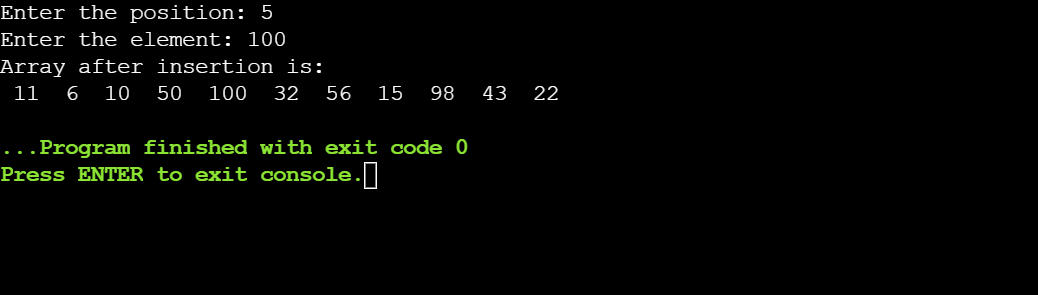In the above example, an element that needs to be inserted is taken as input. The position where this element is to be stored is also taken as input. The array elements are shifted to the right to make space for the new element. After insertion, the size of the array is incremented.

### Example 3: Search an Element in the Array.

#include <stdio.h>

int main()

{

int i, element;

// initialize an array.

int my_array = {11, 6, 10, 50, 32, 56, 15, 98, 43, 22};

printf(“Enter element to be searched:n”);

// input element to be searched.

scanf(“%d”, &element);

// traverse the array to search the element.

for (i = 0; i <= 9; i++)

{

if (my_array[i] == element)

{

// print the index at which

// the element is found.

printf(“Element found at index %d”, i);

break;

}

}

if (i == 10)

{

}

return 0;

}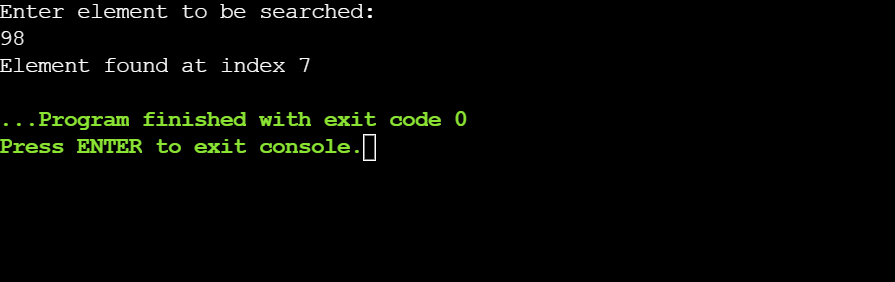In the above example, an element is taken as input from the user. The array is traversed to search this element linearly by comparing all array elements with the element to be found. If the element exists, then its index is printed and the “for loop” is exited. If the element does not exist, then the “for loop” is iterated interrupted from 0 to 9, and “i” will be equal to 10.

### Example 4: Sorting Elements of an Array

#include <stdio.h>

int main()

{

int i, j, temp;

// initialize an array.

int my_array = {11, 6, 10, 50, 32, 56, 15, 98, 43, 22};

// print unsorted array.

printf(“Original array is: n”);

for (i = 0; i < 10; i++)

{

printf(“%d “, my_array[i]);

}

// sort the array elements in descending order.

for (i = 0; i < 10; i++)

{

for (j = i + 1; j < 10; j++)

{

if (my_array[j] > my_array[i])

{

temp = my_array[i];

my_array[i] = my_array[j];

my_array[j] = temp;

}

}

}

// print the sorted elements.

printf(“nnSorted array in descending order is: n”);

for (i = 0; i < 10; i++)

{

printf(“%d “, my_array[i]);

}

return 0;

}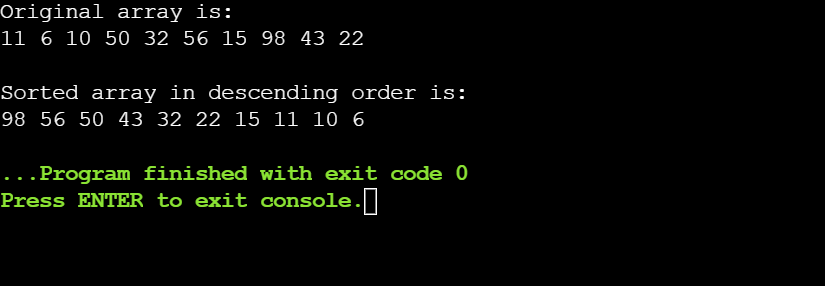In the above example, an array of size 10 is initialized. The array elements are sorted in descending order using the bubble sort algorithm.

### Example 5: Finding the Largest and the Smallest Element in a 1-D Array in C

#include<stdio.h>

int main()

{

// declare the array

int my_array = {11, 6, 10, 50, 32, 56, 15, 98, 43, 22};

int i, smallest, largest;

// assign the value of first array element

// to smallest and largest.

smallest = largest = my_array;

// traverse the array.

for(i = 0; i < 10; i++)

{

// if current element is larger than largest,

// then update the value of largest.

if(my_array[i] > largest)

{

largest = my_array[i];

}

// if current element is smaller than smallest,

// then update the value of smallest.

if(my_array[i] < smallest)

{

smallest = my_array[i];

}

}

// print the smallest and the largest element.

printf(“The smallest element in the array is: %d”, smallest);

printf(“nThe largest element in the array is: %d”, largest);

printf(“nn”);

return 0;

}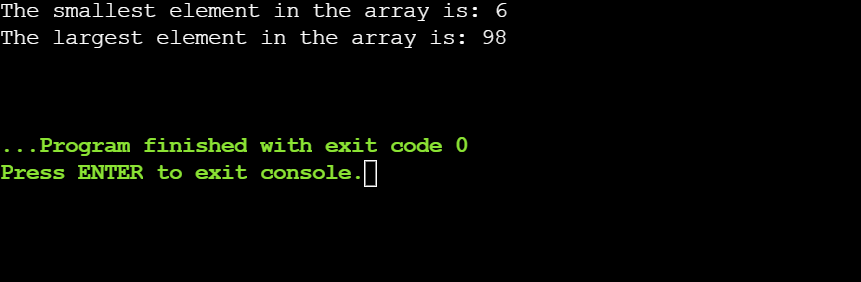In the above program, the largest and the smallest element in an unsorted array is printed. The two variables largest and smallest are created to store the results. These variables are initialized to the first array elements. The array is traversed linearly and each element is compared with the current largest and smallest, and their values are updated if a new largest or smallest element is found.## 2 Dimensional Array in C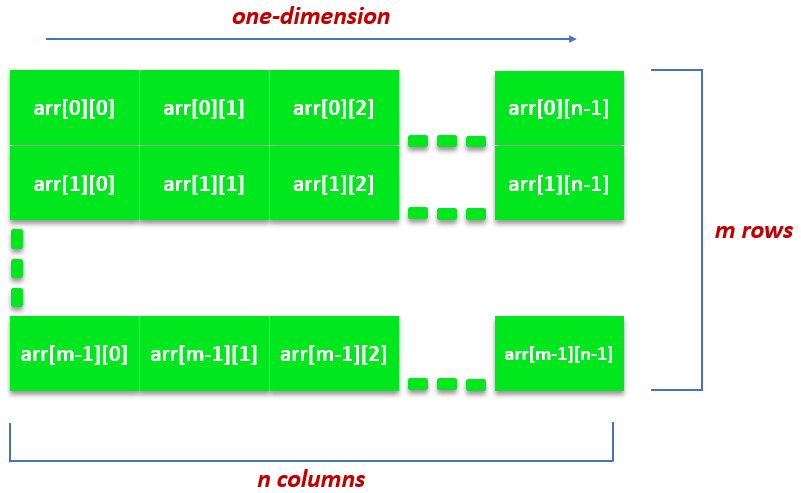A 2-dimensional array or 2-D array is the simplest form of multi-dimensional array in C. Each element in a 2-D array can be represented as a separate 1-D array. A 2-D array contains a certain number of rows and columns and the total number of array elements can be found by multiplying the number of rows and columns. For example, if the array is my_array, then the total number of elements in the array is 6.

A matrix is said to be a square matrix when the number of rows is equal to the number of columns. When the number of rows differs from the number of columns, the matrix is said to be a rectangle matrix. Processing a 2-D array requires a nested loop. The outer loop signifies the number of rows and the inner loop signifies the column of elements of each row. However, the significance of the outer and inner loops can be interchanged depending on whether the user wants a row order matrix or a column order matrix.

## Declaration of 2-D Array in C

### Syntax to Declare a 2-Dimensional Array in C:

// declaring a 2-d array

dataType arrayName[no_of_rows][no_of_columns];

### Description of the syntax:

• dataType: This is the data type that specifies the type of elements to be stored in the array. It can be int, float, double, char.
• arrayName: This is the name of the array. To specify the name of an array, you must follow the same rules which are applicable while declaring a usual variable in C.
• no_of_rows: This is the first dimension of the array. It is an integer specifying the number of rows to be held by the array.
• no_of_columns: This is the second dimension of the array. It’s an integer value representing the number of columns of the array.

### Examples

// 5 x 10 matrix.

int my_array1;

// 3 x 3 square matrix.

float my_array2;

// 2 x 1 matrix.

char my_array3;

Note: The total number of elements that the array can hold is (no_of_rows * no_of_columns). For example, an array arr can have at most 8 elements.

## Initialization of 2-D Array in C

In 1-D arrays, when an array is initialized at the time of its declaration, you can skip specifying its size. However, this scenario is different with 2-D arrays. Only the first dimension can be skipped and the second dimension is mandatory at the time of initialization.

The following ways can be used to initialize a 2-D array in C:

• The conventional way: This is the most common way to initialize an array in C. 1-D arrays equal to the number of the first dimension (or rows) are created having elements equal to the number of the second dimension (or columns).

Example

int my_array = {

{11, 12},

{21, 22},

{31, 32}

};

In the above example, 3 1-D arrays (3 = number of rows) are there with 2 elements each (2 = number of columns).

• The compact way: In this method, all array elements are written in the same line separated by commas. It looks like a 1-D array initialization. This way is not recommended as the initialized array has less readability.

Example

int my_array = {11, 12, 21, 22, 31, 32};

In the above example, all array elements are written inside a single pair of braces “{}”. This type of initialization is less readable.

• Using a loop: Like the 1-D arrays, 2-D arrays can also be initialized using a loop. The most commonly used loop is the “for loop”. Two nested loops are required to initialize the array.

Example

int my_array;

int i, j;

// The first loop runs till the number of rows.

for(i = 0; i < 2; i++)

{

// second loop runs till number of columns.

for (j = 0; j < 3; j++)

{

my_array[i][j] = i + j;

}

}

/* my_array = {

{0, 1, 2},

{1, 2, 3}

} */

In the above example, two “for loops” are used to initialize a 2-D array. The first loop runs from 0 to the number of rows. And the second loop runs from 0 to the number of columns.

## Points to Remember While 2-D Array Initialization

The second dimension is mandatory while declaring a 2-D array, whereas the first dimension can be optional. The following examples illustrate the possible mistakes that can be made while initializing a 2-D array in C:

// valid

int my_array = {10, 20, 30 ,40, 50, 60, 70, 70, 80, 90}

// valid

int my_array[] = {10, 20, 30 ,40, 50, 60, 70, 70, 80, 90}

// invalid: second dimension must be specified.

int my_array[][] = {10, 20, 30 ,40, 50, 60, 70, 70, 80, 90}

// invalid: second dimension must be specified.

int my_array[] = {10, 20, 30 ,40, 50, 60, 70, 70, 80, 90}

## Examples of 2-D Array in C

The following examples illustrate the basic implementation of a 2-D array including input and output operations in a 2-D matrix and finding the transpose of a matrix.

### Example 1: 2-D Array Declaration, Input, and Output.

#include <stdio.h>

int main()

{

// declaring and initializing the 2-D array.

// 2-D array with 5 rows and 2 columns.

int x = {{0, 1}, {2, 3}, {4, 5}, {6, 7}, {8, 9}};

int i, j;

// print the value of each array element.

// outer loop- for rows.

for (i = 0; i < 5; i++)

{

// inner loop- for columns.

for (j = 0; j < 2; j++)

{

printf(“Element at x[ %d”, i);

printf(“][ %d”, j);

printf(“] :”);

printf(“%d”, x[i][j]);

printf(“n”);

}

}

return 0;

}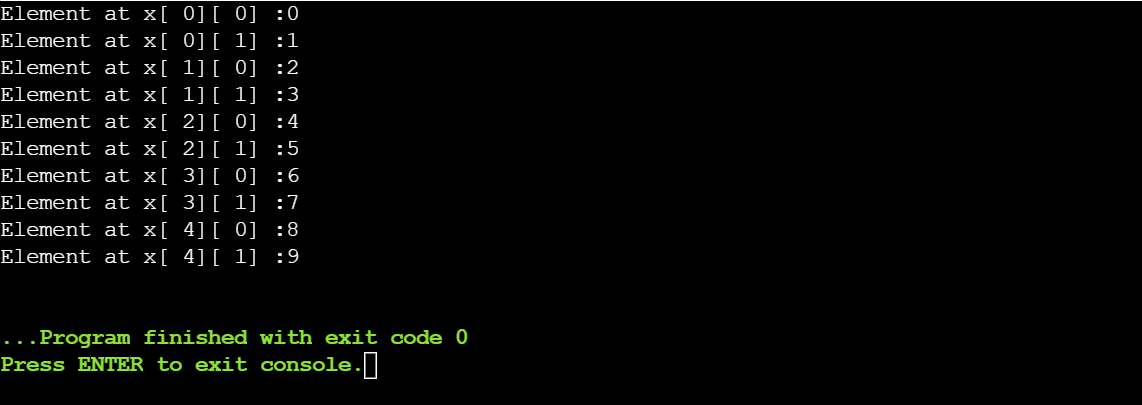In the following example, a 2-D array of 5 rows and 2 columns has been declared and initialized. For printing, we are using two “for loops”. The outer “for loop” is printing the rows while the inner “for loop” is printing columns for every row.

### Example 2: Finding Sum and Product of Two 2 Matrices

#include <stdio.h>

int main()

{

// declaring arr1 and arr2.

int arr1, arr2;

int sum, product;

// reading input for arr1 from the user.

printf(“Enter the elements of arr1 : n”);

for (int i = 0; i < 2; i++)

{

for (int j = 0; j < 2; j++)

{

printf(“arr1[%d][%d] :”, i, j);

scanf(“%d”, &arr1[i][j]);

}

printf(“n”);

}

// reading input for arr2 from the user.

printf(“Enter the elements of arr2: n”);

for (int i = 0; i < 2; i++)

{

for (int j = 0; j < 2; j++)

{

printf(“arr2[%d][%d] :”, i, j);

scanf(“%d”, &arr2[i][j]);

}

printf(“n”);

}

// adding the corresponding array elements.

printf(“Sum array is : n”);

for (int i = 0; i < 2; i++)

{

for (int j = 0; j < 2; j++)

{

sum[i][j] = arr1[i][j] + arr2[i][j];

//print the sum array

printf(“%dt”, sum[i][j]);

}

printf(“n”);

}

// multiplying the corresponding array elements.

printf(“Product array is : n”);

for (int i = 0; i < 2; i++)

{

for (int j = 0; j < 2; j++)

{

product[i][j] = arr1[i][j] * arr2[i][j];

// print the product array.

printf(“%dt”, product[i][j]);

}

printf(“n”);

}

return 0;

}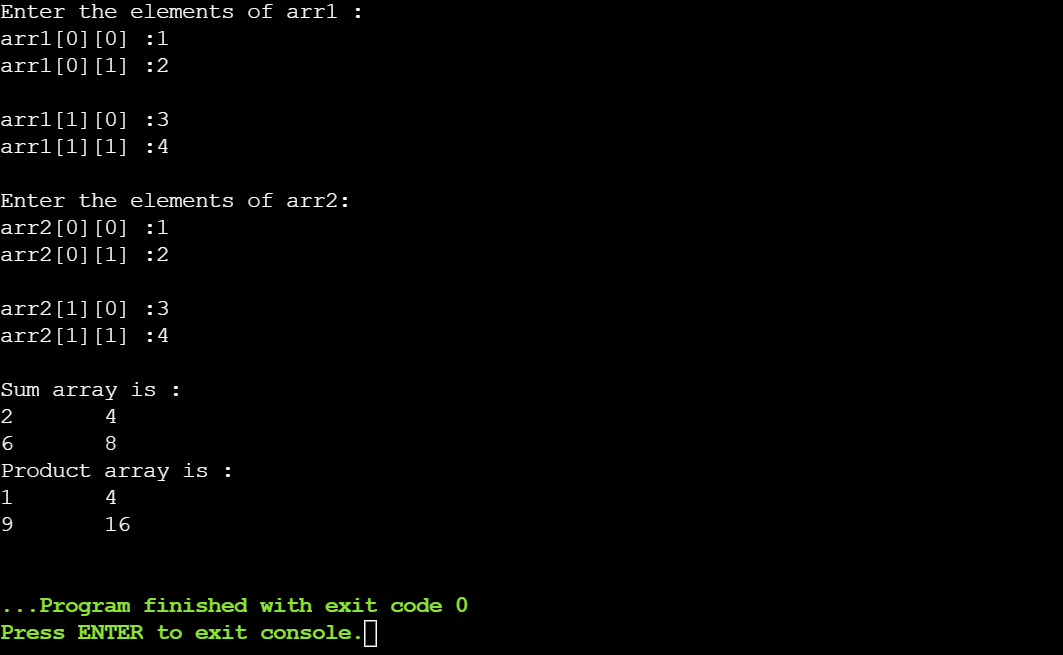For finding the addition of two matrices, the number of rows and columns should be the same in both the matrices. And for finding the product of two matrices, the number of columns in the first matrix should be the same as the number of rows in the second matrix.

To get the sum of the two matrices, a resultant matrix of the same order is declared. To get the product of the two matrices, a resultant matrix having rows equal to the first matrix and columns equal to the second matrix is declared.

### Example 3: Transpose of a Matrix

#### Square Matrix

#include <stdio.h>

int main()

{

// declaring the matrices.

int arr, transpose;

// reading the input from the user.

printf(“Enter elements of the arrn”);

for (int i = 0; i < 2; i++)

for (int j = 0; j < 2; j++)

scanf(“%d”, &arr[i][j]);

// copy the array element into another element.

for (int i = 0; i < 2; i++)

for (int j = 0; j < 2; j++)

transpose[j][i] = arr[i][j];

printf(“Transpose of the arr:n”);

//print the transpose of the arr

for (int i = 0; i < 2; i++)

{

for (int j = 0; j < 2; j++)

printf(“%dt”, transpose[i][j]);

printf(“n”);

}

return 0;

}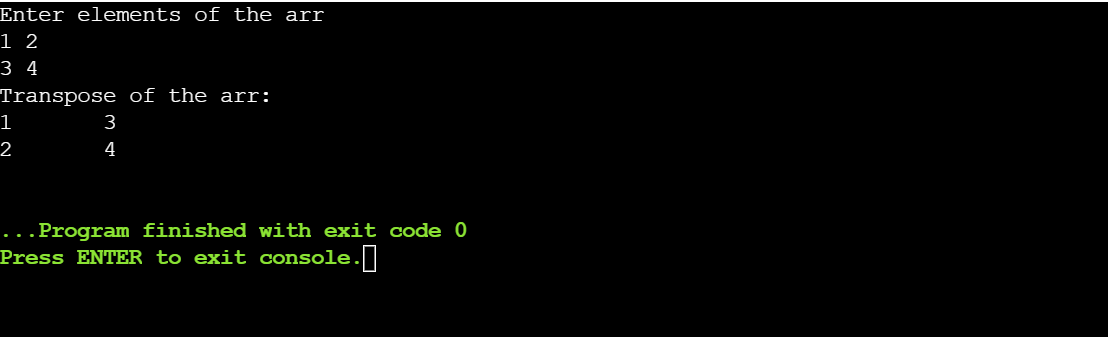In the above example, the transpose of a square matrix is printed. For printing the transpose of the matrix, first, the matrix elements are copied in another matrix and then the rows and the columns of the copied matrix are reversed.

#### Rectangular Matrix

#include <stdio.h>

int main()

{

// declaring the matrices.

int arr, transpose;

// reading the input from the user.

printf(“Enter elements of the arrn”);

for (int i = 0; i < 2; i++)

{

for (int j = 0; j < 3; j++)

scanf(“%d”, &arr[i][j]);

}

// copy the array element into another element.

for (int i = 0; i < 2; i++)

{

for (int j = 0; j < 3; j++)

transpose[j][i] = arr[i][j];

}

printf(“Transpose of the arr:n”);

// print the transpose of the array.

for (int i = 0; i < 3; i++)

{

for (int j = 0; j < 2; j++)

printf(“%dt”, transpose[i][j]);

printf(“n”);

}

return 0;

}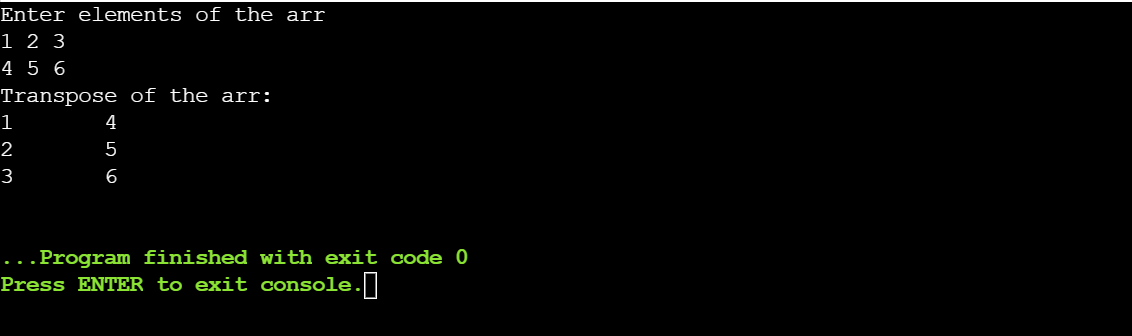In the above example, the matrix is a rectangle matrix which means that the number of the rows is not equal to the number of the columns. The logic is the same as that in the previous example.

### Example 4: Check if the Given Matrix is Sparse or Not

#include <stdio.h>

#include <stdlib.h>

int main()

{

int row, col, a, count = 0;

// read the number of rows and columns from the user.

printf(“Enter the number of rows: n”);

scanf(“%d”, &row);

printf(“Enter the number of columns: n”);

scanf(“%d”, &col);

// read the elements of the matrix from the user.

printf(“Enter the matrix elements: n”);

for (int i = 0; i < row; i++)

{

for (int j = 0; j < col; j++)

{

scanf(“%d”, &a[i][j]);

}

}

// print the matrix.

printf(“Matrix is:n”);

for (int i = 0; i < row; i++)

{

for (int j = 0; j < col; j++)

{

printf(“%dt”, a[i][j]);

}

printf(“n”);

}

// check if the matrix is sparse or not.

for (int i = 0; i < row; i++)

{

for (int j = 0; j < col; j++)

{

if (a[i][j] == 0)

count++;

}

}

if (count > ((row * col) / 2))

printf(“Matrix is a sparse matrix n”);

else

printf(“Matrix is not sparse matrixn”);

}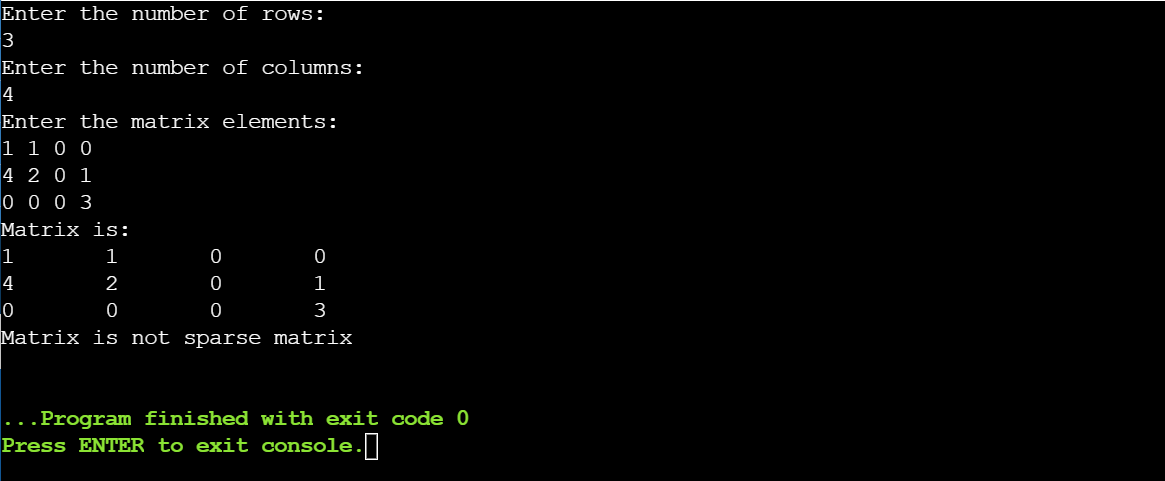In the above example, it is checked whether the matrix is sparse or not. A sparse matrix is the one in which the number of 0’s is greater than the non-zero elements. A counter is maintained to count the number of 0’s. At last, the count of 0’s is compared with half the total number of elements of the matrix.

## Arrays vs. Pointers

Arrays can behave like pointers in many circumstances such as when an array is passed to function, it is treated as a pointer. Also, pointer arithmetic is applicable to arrays. However, these two differ from each other in many ways. The following comparison chart summarizes the key differences between a pointer and an array in C.

### POINTER

DEFINITION

An array stores one or more values of a similar type.

A pointer stores the address or memory location of a variable.

SYNTAX

type array_name[size];

Type *ptr_name;

MEMORY ALLOCATION

Contiguous or sequential memory is allocated to the elements of an array.

A pointer can be allocated to any random available memory.

MEMORY SPACE

Arrays are static, so their size cannot be altered.

A pointer is dynamic, so the memory size can be either altered or even freed.

NO. OF VALUES STORED

A single array can store a large number of elements.

A pointer can point to only one variable’s address.

TYPE

The data type of the array is determined by the type of elements stored in it.

The data type of a pointer is determined by the type of the variable whose memory location it is pointing to.

Sizeof() Operator

When an array is passed to the sizeof() operator, the combined size of all the stored elements is returned.

When a pointer is passed to the sizeof() operator, the size of the pointer is printed (generally 8). This size is the same for any type of pointer.

COMPILE-TIME/ RUN-TIME

Memory is allocated to an array during the compilation.

Memory is allocated to a pointer during the run time of the program.

## Passing an Array to a Function

When an array in C is passed to a function, only the address of the array’s first element is passed to the function. And being contiguous in nature, the whole array can be accessed by the function using the address of the first element.

### Passing an Array Element to a Function

We can pass a  single array element to a function as its argument. The following examples illustrate how we can pass an array element to a function. Here, we have made a function that is simply printing the element passed to it.

#include<stdio.h>

//function having an int type parameter

void func(int a)

{

printf(“Array element passed to the function is: %d”, a);

}

int main()

{

//initialized 1-D array

int arr[] = { 1, 2, 3, 4, 5 };

//function call

func(arr);        //passing arr i.e., 3 to the func.

return 0;

}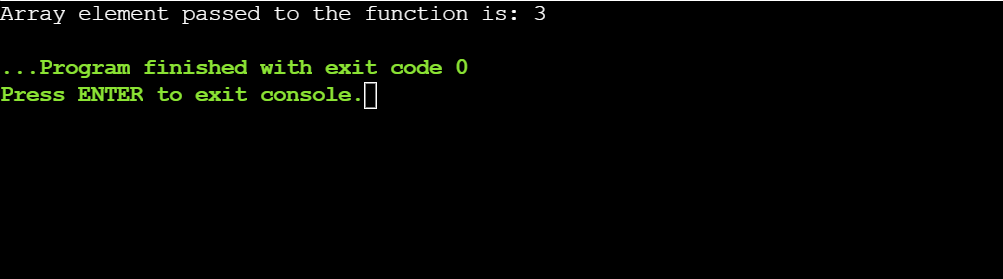### Passing a 1-D Array to a Function

In the above section, we saw passing an array element to a function. In this section, we are going to have a look at how a 1-D array can be passed to a function through a simple example given below. Here a function has been made to which we are passing an array and its size and the function is returning the maximum element of that array.

#include<stdio.h>

// function find the greatest array element

int maximum(int arr[], int size)

{

int i;

int large = arr;

for(i = 0; i<size; i++)

{

if(arr[i]>large)

{

large = arr [i];

}

}

return large;

}

int main()

{

int result;

// 1-D array initialization

int arr[] = {100, 2, 1, 120, 55, 41};

int size = sizeof(arr) / sizeof(int);

// function call

result = maximum(arr, size);   // 1-D array is passed to the function.

printf(“The greatest array element is: %d”, result);

return 0;

}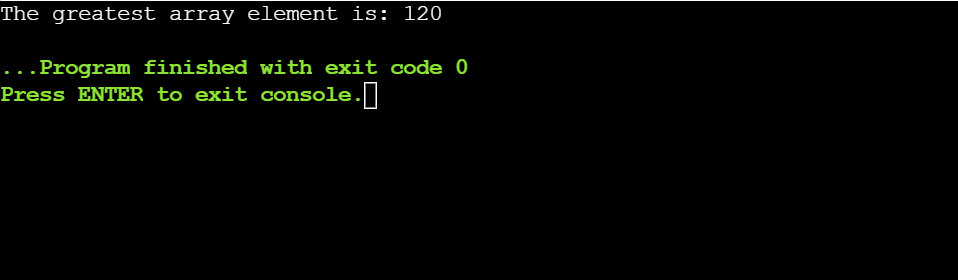### Passing a Multidimensional Array to a Function

We can pass a matrix or a 2-D array to a matrix as well. The below example illustrates the same where a matrix is passed to a function and the function is printing that matrix.

#include<stdio.h>

// function to print the matrix elements

void printMatrix(int arr)

{

int i, j;

printf(“The Matrix thus formed is: n”);

for (i = 0; i < 3; ++i)

{

// change the line

printf(“n”);

for (j = 0; j < 3; ++j)

{

printf(“%dt”, arr[i][j]);

}

}

}

int main()

{

int arr, i, j;

printf(“Enter the elements: n”);

for (i = 0; i < 3; ++i)

{

for (j = 0; j < 3; ++j)

{

scanf(“%d”, &arr[i][j]);

}

}

// passing 2-D array or a

// Matrix as an argument

printMatrix(arr);

return 0;

}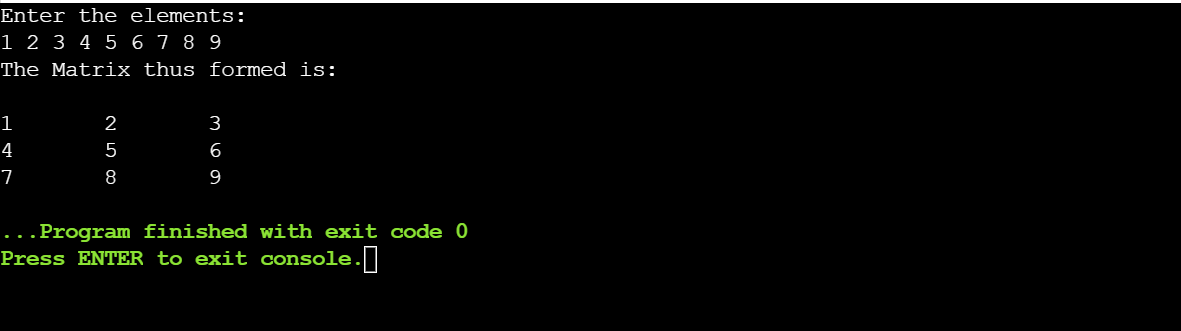## Pointers to Array

As we have already discussed in the earlier section the relationship and difference between arrays and pointers in C. In this section, we are going to discuss how a pointer can be declared to store the address of an array.

The following advantages are achieved by declaring a pointer to an array:

• The array elements can now be accessed in multiple ways.
• The time and space complexity of the program can be reduced.
• Arrays can be manipulated more efficiently.

#include<stdio.h>

int main()

{

int i;

int my_array = {10, 20, 30, 40, 50, 60, 70, 80};

// declare a pointer pointing to the above array.

int *my_ptr = my_array;

my_ptr = my_array;

// access an array element using the pointer.

*(my_ptr + 1) = 100;

// print array elements using the pointer.

printf( “Array elements are: n”);

for ( i = 0; i < 8; i++ ) {

printf(“*(my_ptr + %d) = %dn”,  i, *(my_ptr + i) );

}

return 0;

}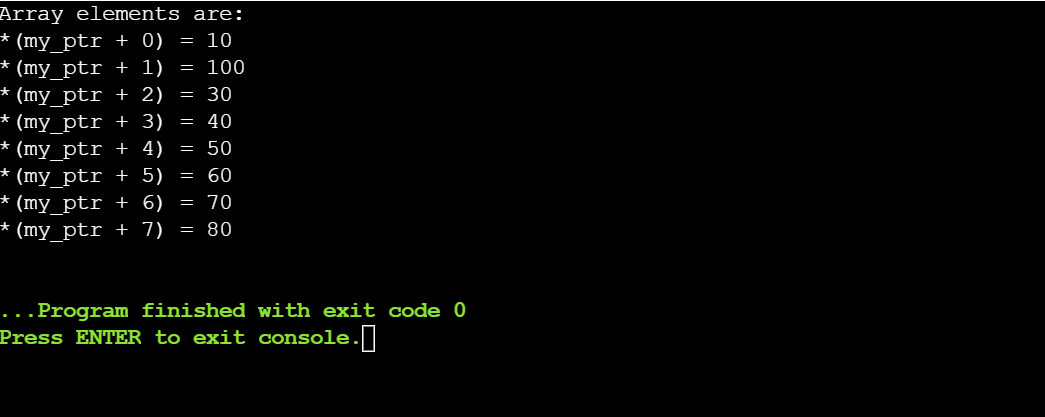In the above program, a pointer *my_ptr is pointing to the array my_array. This simply means that the address of the array’s first element (i.e. my_array) is stored in the pointer. The pointer now has access to all elements of the array.

That was all about Array in C

Accelerate your career as a skilled MEAN Stack Developer by enrolling in a unique Full Stack Web Developer – MEAN Stack Master’s program. Get complete development and testing knowledge on the latest technologies by opting for the MEAN Stack Developer Course. Contact us TODAY!

## Final Thoughts!

To sum up, in this article you have learned the concept of array in C. You started with a brief introduction to the array data structure and gradually moved ahead to discuss the need, advantages, and disadvantages of arrays. Next, you saw the different ways to declare and initialize arrays in C.

Next, you learned how to access array elements and input or output elements to/from an array. Moving ahead, you saw some examples of 1D and 2D arrays. Finally, you learned how to pass an array to a function, a concept called pointers to an array, and the differences between an array and a pointer.

Why stop here? If you want to learn full-stack web development, you should definitely check out Simplilearn’s 9-month instructor-led certification training course on Full Stack Web Development. This will get you started in professional web development and you will be able to learn advanced concepts such as Agile methodologies, DevOps practices, Java and its frameworks such as Spring, Hibernate, etc., JS, CSS, HTML, etc. If you have a knack for learning new courses, you should definitely check out Simplilearn’s complete list of free courses.

If you have any queries in this “Array in C” article or suggestions for us, please mention them in the comment box and our experts answer them for you as soon as possible.

Happy Learning!

https://www.simplilearn.com/tutorials/c-tutorial/array-in-c